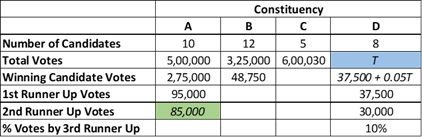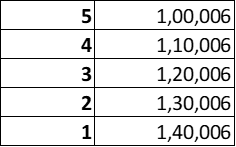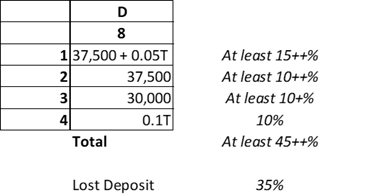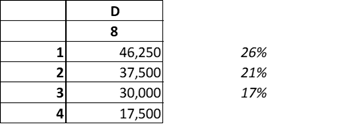# In an election several candidates contested for a constituency - CAT DILR questions – Data Interpretation

## CAT 2020 - Slot 2 - Question 3 - In an election several candidates contested for a constituency

Q13. What is the percentage of votes polled in total by all the candidates who lost their security deposits while contesting for constituency A? (TITA)

13. 9.

Let’s try to fill the table first. Partially applying points 1 and 3 we can get the votes for the 2nd runner up in A and the winning candidate in D.To begin answering this question, we need to first see what is the% of total votes between the candidates we already know about: Winner + 1st + 2nd Runner Up total: 455,000 (91%)
So the vote share among the remaining 7 candidates was only 9%
To lose security deposit, one must get less than or equal to 1/6th i.e. lesser than 16.67% of the total votes. Clearly that is indeed the case with the remaining candidates in A.

Q14. How many candidates who contested in constituency B lost their security deposit?

14. 11.

The winning candidate in B had a 15% vote share. Meaning everyone else (the other 11) had a less than 16.67% vote share as well so they all will lose their security deposits.

Q15. What BEST can be concluded about the number of votes polled by the winning candidate in constituency C?

a. between 1,40,005 and 1,40,010

b. 1,40,006

c. less than 2,00,010

d. 1,40,010

15. B.

It is mentioned that none in C lost their security deposit and the delta in votes was at least 10,000

The least possible vote share is slightly more than 1/6th, meaning the lowest possible value here is: 600,030 / 6 +1 = 100,005 + 1. If noone lost the security deposit, then the least possible value is 100,006

Assuming a 100,00 vote delta just for the time being we get the following as our possible valuesClearly given the constraints, there isn’t too much we can do to flex the numbers here.This is the only possible way to satisfy the constraints. So this must be the answer.

16. What was the number of valid votes polled in constituency D?

a. 1,75,000

b. 62,500

c. 1,25,000

d. 1,50,000

16. A.

This is how the table looks for D as of now:Check using the options. Clearly it cannot be 62,500 (Option C) because the total of 2, 3 adds up to a number greater than that

A: 175,000

The table looks like the following:In this option, clearly the top 3 each have more than 16.67% and the rest have 35%. This option satisfies the constraints and hence is the correct answer.

Q17. The winning margin of a constituency is defined as the difference of votes polled by the winner and that of the first runner up. Which of the following CANNOT be the list of constituencies, in increasing order of winning margin?

a. B, D, C, A

b. D, B, C, A

c. D, C, B, A

d. B, C, D, A

17. D.

A clearly has the highest margin

With B, margin could be as low as possible since everyone lost their deposit. Highest could be as follows: assume that the first 11 people got 1 – 11 votes only (since there are no ties, we are increasing the numbers by 1 for each position). So the winning margin can be as high as ~48,000

C it is 10,000 as calculated earlier

D is less than 10,000 for sure since it is 5% of the valid votes

So D cannot be more than C. The only option which has D more than C is the last one

Q18. For all the four constituencies taken together, what was the approximate number of votes polled by all the candidates who lost their security deposit expressed as a percentage of the total valid votes from these four constituencies?

a. 32.00%

b. 38.25%

c. 23.54%

d. 23.91%

18. D.

Just calc using available data

For A: Votes that count towards a deposit loss=> 500,000 – (275,000 – 95,000 – 85,000) = 45,000

For B: All except the winner lost, i.e. =>325,000 – 48,750 = 276,250

For C: Noone lost

For D: 35% of the total i.e. 35% of 175,000 => 61,250

Answer: (45,000 + 276,250 + 61,250) / (500,000 + 325,000 + 600,003 + 175,000)

### Past Year Question Paper & SolutionsCounselling Session
By IIM Mentor

#### Free Material Area